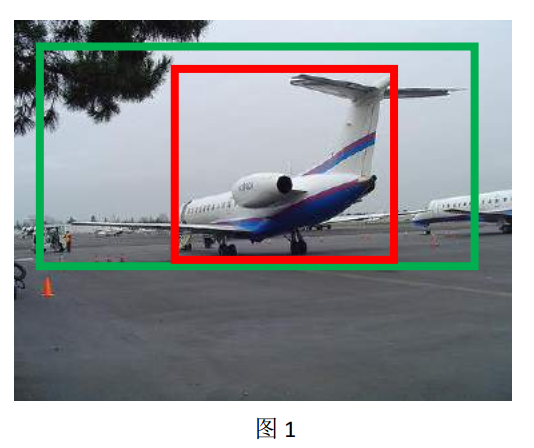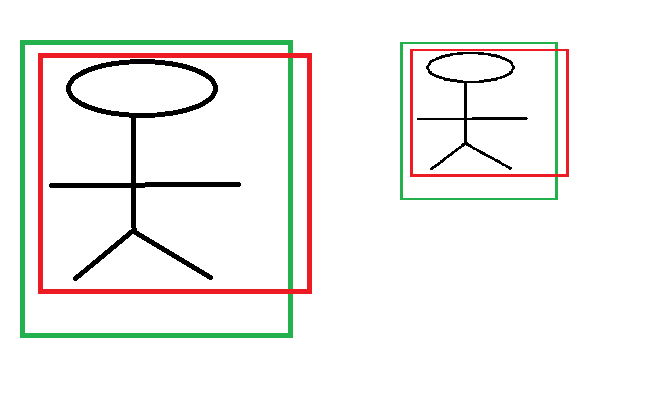# Bounding-Box regression

• 为什么要边框回归？
• 什么是边框回归？
• 边框回归怎么做的？
• 边框回归为什么宽高，坐标会设计这种形式？
• 为什么边框回归只能微调，在离Ground Truth近的时候才能生效？

## 为什么要边框回归？## 边框回归是什么？## 边框回归怎么做的？

1. 先做平移(Δx,Δy)， Δx=Pwdx(P),Δy=Phdy(P) 这是R-CNN论文的：
G^x=Pwdx(P)+Px,(1)
G^y=Phdy(P)+Py,(2)
2. 然后再做尺度缩放(Sw,Sh)Sw=exp(dw(P)),Sh=exp(dh(P)), 对应论文中：
G^w=Pwexp(dw(P)),(3)
G^h=Phexp(dh(P)),(4)

### Input:

RegionProposalP=(Px,Py,Pw,Ph)，这个是什么？ 输入就是这四个数值吗？其实真正的输入是这个窗口对应的 CNN 特征，也就是 R-CNN 中的 Pool5 feature（特征向量）。 (注：训练阶段输入还包括 Ground Truth， 也就是下边提到的t=(tx,ty,tw,th))

### Output:

tx=(GxPx)/Pw,(6)

ty=(GyPy)/Ph,(7)

tw=log(Gw/Pw),(8)

th=log(Gh/Ph),(9)

Loss=iN(tiw^Tϕ5(Pi))2

W=argminwiN(tiw^Tϕ5(Pi))2+λ||w^||2

## 为什么宽高尺度会设计这种形式？## 为什么IoU较大，认为是线性变换？

Log函数明显不满足线性函数，但是为什么当Proposal 和Ground Truth相差较小的时候，就可以认为是一种线性变换呢？大家还记得这个公式不？参看高数1。

limx=0log(1+x)=x

tw=log(Gw/Pw)=log(Gw+PwPwPw)=log(1+GwPwPw)

## 总结